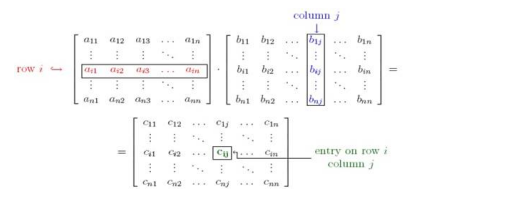# Solve this following

Question:

Compute $A B$ and $B A$, which ever exists when

$A=\left[\begin{array}{rrr}0 & 1 & -5 \\ 2 & 4 & 0\end{array}\right]$ and $B=\left[\begin{array}{cc}1 & 3 \\ -1 & 0 \\ 0 & 5\end{array}\right]$

Solution:

Given : $A=\left[\begin{array}{rrr}0 & 1 & -5 \\ 2 & 4 & 0\end{array}\right]$ and $B=\left[\begin{array}{cc}1 & 3 \\ -1 & 0 \\ 0 & 5\end{array}\right]$

Matrix A is of order $2 \times 3$ and Matrix $B$ is of order $3 \times 2$

To find : matrices $A B$ and $B A$

Formula used :Where $c_{i j}=a_{i 1} b_{1 j}+a_{i 2} b_{2 j}+a_{i 3} b_{3 j}+\ldots \ldots \ldots \ldots \ldots . .+a_{i n} b_{n j}$

If $A$ is a matrix of order $a \times b$ and $B$ is a matrix of order $c \times d$, then matrix $A B$ exists and is of order $a \times d$, if and only if $b=$ $c$

If $\mathrm{A}$ is a matrix of order $\mathrm{a} \times \mathrm{b}$ and $\mathrm{B}$ is a matrix of order $\mathrm{c} \times \mathrm{d}$, then matrix $\mathrm{BA}$ exists and is of order $\mathrm{c} \times \mathrm{b}$, if and only if $d=$ a

For matrix $\mathrm{AB}, \mathrm{a}=2, \mathrm{~b}=3, \mathrm{c}=3, \mathrm{~d}=2$, matrix $\mathrm{AB}$ exists and is of order $2 \times 2$,as

$b=c=3$

Matrix $A B=\left[\begin{array}{ccc}0 & 1 & -5 \\ 2 & 4 & 0\end{array}\right] \times\left[\begin{array}{cc}1 & 3 \\ -1 & 0 \\ 0 & 5\end{array}\right]=\left[\begin{array}{cc}0(1)+1(-1)-5(0) & 0(3)+1(0)-5(5) \\ 2(1)+4(-1)+0(0) & 2(3)+4(0)+0(5)\end{array}\right]$

Matrix $A B=\left[\begin{array}{cc}0-1-0 & 0+0-25 \\ 2-4+0 & 6+0+0\end{array}\right]=\left[\begin{array}{cc}-1 & -25 \\ -2 & 6\end{array}\right]$

Matrix $A B=\left[\begin{array}{cc}-1 & -25 \\ -2 & 6\end{array}\right]$

Matrix $A B=\left[\begin{array}{cc}-1 & -25 \\ -2 & 6\end{array}\right]$

For matrix $\mathrm{BA}, \mathrm{a}=2, \mathrm{~b}=3, \mathrm{c}=3, \mathrm{~d}=2$, matrix BA exists and is of order $3 \times 3$, as

$d=a=2$

Matrix BA $=\left[\begin{array}{cc}1 & 3 \\ -1 & 0 \\ 0 & 5\end{array}\right] \times\left[\begin{array}{ccc}0 & 1 & -5 \\ 2 & 4 & 0\end{array}\right]=\left[\begin{array}{ccc}1(0)+3(2) & 1(1)+3(4) & 1(-5)+3(0) \\ -1(0)+0(2) & -1(1)+0(4) & -1(-5)+0(0) \\ 0(0)+5(2) & 0(1)+5(4) & 0(-5)+5(0)\end{array}\right]$

Matrix BA $=\left[\begin{array}{ccc}0+6 & 1+12 & -5+0 \\ 0+0 & -1+0 & 5+0 \\ 0+10 & 0+20 & 0+0\end{array}\right]$

Matrix BA $=\left[\begin{array}{ccc}6 & 13 & -5 \\ 0 & -1 & 5 \\ 10 & 20 & 0\end{array}\right]$

Matrix $B A=\left[\begin{array}{ccc}6 & 13 & -5 \\ 0 & -1 & 5 \\ 10 & 20 & 0\end{array}\right]$